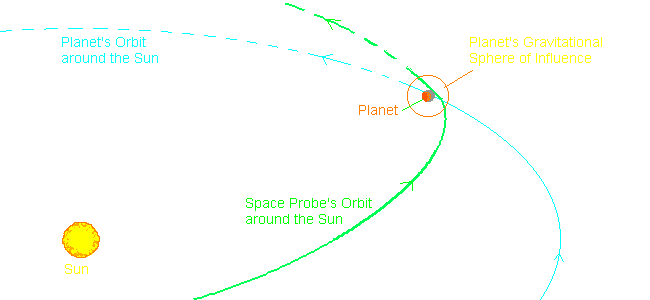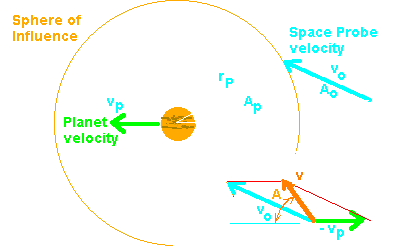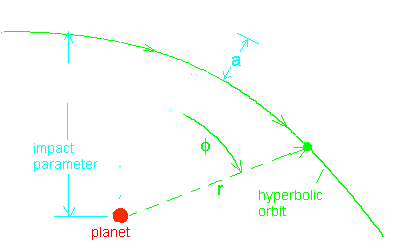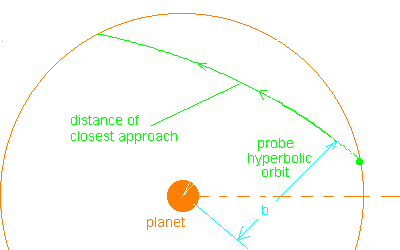## Satellite Orbits - Gravitational Assist from Planets

### by Larry Bogan#### General Description

Kepler's Laws apply to elliptical orbits involving only two bodies. When three bodies are involved we have to make some changes and approximations if we wish to use simple orbital mechanics. In the case shown below, a space probe is sent into orbit around the Sun heading toward the planet. This orbit follows an elliptical orbit around the Sun. The planet does not interact with the probe until it approaches close enough for the planet's gravity to be stronger than that of the Sun's. This region is called the "Sphere of Influence".
Inside this region, the probe is in orbit around the planet rather than the Sun. The probe entered the sphere of influence with more than the escape velocity of the planet, and as a result will escape the sphere of influence. While near the planet the path will be a hyperbolic one rather than elliptical and its center of motion will be the planet rather than the Sun. Once the probe leave the planet's sphere of influence it will again be in an elliptical orbit around the Sun - but different from that when it approached the planet.

We will show what is happening by considering the following:
1. Gravitational Sphere of Influence of a planet
2. Relative Motion of the space probe and the planet
3. Hyperbolic Orbits around the planet
4. Transitions from Solar orbit to Planetary orbits back to a Solar orbit

### Gravitational Sphere of Influence

The Gravitational Force varies in proportion to the Mass of the attracting body and inversely with the square of the distance to the body. The Sun is much more massive than any of the planets and its gravity dominates the Solar System. Only quite near the planets, does the planetary gravity become stronger than that of the Sun.The first reaction is to determine the point between Sun and Planet where their gravitational forces cancel out. This would be OK if we were not considering a moving objects. Since there is orbit acceleration as well as a gravitational one, these have to be considered also. Laplace derived the following for the Radius of the Sphere of Influence

rP = DSP [MP/MSun]2/5

Where
• DSP = the distance between the Sun and the Planet
• MP = mass of the planet
• MSun = mass of the Sun

#### Example: Jupiter and Earth

Jupiter:

The Sun is 1047 times more massive than Jupiter (MSun/MJ = 1047). The distance between the two is 5.02 AU (AU = Astronomical Units = 150 million km). So
rJ = (5.2 x 1.5x108km) [1/1047]2/5 = 48.3 million km
The most distant satellites of Jupiter are about 1/2 this distance away from the planet. Jupiter's radius is 73,500 km
so in terms of the planets radius, RJupiter: rJ = 657 RJupiter

Earth:
Earth is 1/333,000 as massive as the Sun and only 1 AU away. It's sphere of influence is thus:
rE = 1.5x108km [1/333,000]2/5 = 927,000 km
= 2.4 x distance from Earth to the Moon
= 145 REarth (145 times the radius of the Earth)

### Relative MotionIn order to properly describe the motion of the probe with in the sphere of influence of the planet, we must know its velocity with respect to the planet. The motion in the solar sysem is known relative to the Sun and we must transform the velocity relative to the Sun, vo to one relative to the planet, v. We must know the velocity of the planet, vp, relative to the sun. We must also know where the space probe enters the sphere of influence. The diagram to the right illustrates the parameters involved.
The velocity relative to the planet is given by subtracting the planet's velocity from the space probe's.

v = vo - vp

Since velocity has direction and magnitude, we need to determine both. The result of a little trigonometry gives
vx = vp - vo cos Ao
vy = vo sin Ao
The angle of the new velocity is
tan(A) = vy/vx
The magnitude of the velocity is then determined using the components
| v | = [vp2 + vo2 - 2 vp vo cos Ao]1/2

### Hyperbolic OrbitsSince the space probe enters the sphere of influence with a net velocity inward, the probe will have a velocity relative to the planet greater than the escape velocity of the planet. This produces a hyperbolic orbit within the sphere of influence. All orbits (elliptical, parabolic, and hyperbolic) are described geometrically by,

r = a ( e2 - 1) /( e cos f + 1)

Where
r = distance from the center of the planet at angle f
e = eccentricity of the orbit ( e > 1 for a hyperbolic orbit)
a = semi-major axis for elliptical orbit,
distance from closest approach to the intersection of the asymptotic lines for hyperbolic orbits
f = position angle relative to perihelion

When the probe is very far from the planet (entering the sphere of influence), then it is at its maximum angle , fm.
e cos fm + 1 = 0
and this gives a relationship with the eccentricity of the orbit.
e = -1/cos fm
The impact parameter, b, is the distance from the planet that the probe would have it travelled in a straight line inside the sphere of influence. This parameter along with the velocity, v, determines the deflection angle, 2q.

b = [GM/v2 ] cot q

M = mass of the planet
G = Universal Gravitation constant = 6.67x10-11 N kg2/m2
The maximum angle and the deflection angle are related by
fm = p/2 + q
Hence the eccentricity is e = 1/sin q

These relationships will be important in deternining the effect of the planet on the space probes orbit. One useful property of the orbits is that they are symmetric about the semi-major axis. The velocity of infall before perijov (closest point of approach to Jupiter) will have the same magnitude as the outgoing velocity at the same distance from perijov. We will use this in our example.

### Putting it all together: Gravitational Assist for a Space ProbeLet's set up a realistic example of using Jupiter to increase the energy of a space probe as it flys by Jupiter.
We will have to do this in several separate steps in order to not get to confused.

1. Establish the orbit and its parameters for the flight of the space probe from Earth to Jupiter.
Earth to Jupiter Orbit
2. Select the point where the probe enters the sphere of influence of Jupiter and transform the space probe velocities to those relative to Jupiter.
Velocity Transform
3. Determine the hyperbolic orbit of the probe near Jupiter.
4. Transform the velocity of the hyperbolic orbit on leaving the sphere of influence to a Solar system velocity.
5. Determine the new orbit of the space probe around the Sun.
6. Tisserand's Criteria is a requirement that conservation of energy and momentum hold during the flyby of a Planet. In this page we show that indeed it does and is a useful method of determining the final orbit.